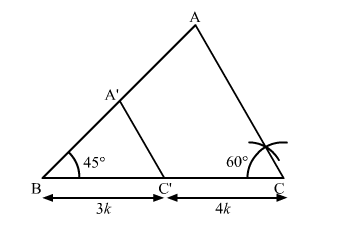# To construct a triangle similar to ∆ABC in which BC = 4.5 cm,`
Question:

To construct a triangle similar to $\triangle \mathrm{ABC}$ in which $\mathrm{BC}=4.5 \mathrm{~cm}, \angle \mathrm{B}=45^{\circ}$ and $\angle \mathrm{C}=60^{\circ}$, using a scale factor of $\frac{3}{7}, \mathrm{BC}$ will be divided in the ratio

(a) $3: 4$

(b) $4: 7$

(c) $3: 10$

(d) $3: 7$

Solution:

To construct a triangle similar to $\triangle \mathrm{ABC}$ in which $\mathrm{BC}=4.5 \mathrm{~cm}, \angle \mathrm{B}=45^{\circ}$ and $\angle \mathrm{C}=60^{\circ}$, using a scale factor of $\frac{3}{7}, \mathrm{BC}$ will be divided in the ratio $3: 4$.Here, ∆ABC ∼ ∆A'BC'

BC' : C'C = 3 : 4

or BC' : BC = 3 : 7

Hence, the correct answer is option A.